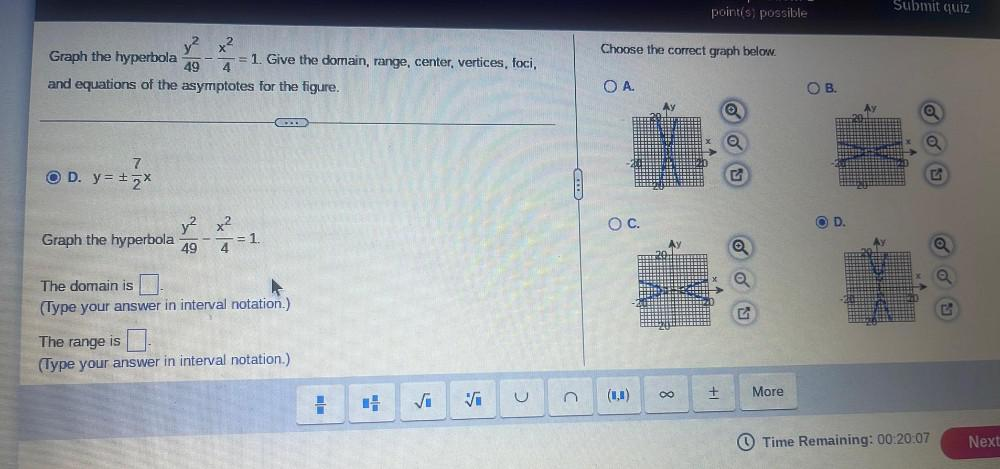Question:

# pointis, possible Submit quiz Choose the correct graph below. y2x2 Graph the hyperbola = 1. Give the domain, range, center, vertpointis, possible Submit quiz Choose the correct graph below. y2x2 Graph the hyperbola = 1. Give the domain, range, center, vertices, foci, 49 4 and equations of the asymptotes for the figure. OA. OB. Ay O O 7 OD. y= + 2* OC. OD y2 x2 Graph the hyperbola 49 4 =1 The domain is (Type your answer in interval notation.) The range is (Type your answer in interval notation.) S. u (1,1) VI More + oo C . O Time Remaining: 00:20:07 Next